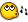## Recommended Posts

I really am not having luck searching google so i think i'll ask you cuys. Whats the formula to fin the angle that 3 points make... like say i have point A, B, and C somewhere on my desktop... how can i determine the angles of them? thanks in advance.

##### Share on other sites

I really am not having luck searching google so i think i'll ask you cuys. Whats the formula to fin the angle that 3 points make... like say i have point A, B, and C somewhere on my desktop... how can i determine the angles of them? thanks in advance.

Whipped up this UDF, i think it works... but its been a while since i tried trig..

Uses the '_Distance' UDF from SolidSnake

```Func _Angle(\$xa, \$ya, \$xb, \$yb, \$xc, \$yc, \$angle = "B")
\$SideA = _Distance(\$xc, \$yc, \$xb, \$yb)
\$SideC = _Distance(\$xa, \$ya, \$xb, \$yb)
\$SideB = _Distance(\$xc, \$yc, \$xb, \$yb)
Switch \$angle
Case "B"
Return ACos((\$SideA^2 + \$SideC^2 - \$SideB^2)/(2*\$SideA*\$SideC))
Case "A"
Return ACos((\$SideB^2 + \$SideC^2 - \$SideA^2)/(2*\$SideB*\$SideC))
Case "C"
Return ACos((\$SideA^2 + \$SideB^2 - \$SideC^2)/(2*\$SideA*\$SideB))
EndSwitch
EndFunc

Func _Distance(\$iX1, \$iY1, \$iX2, \$iY2)
Return Sqrt((\$iX1 - \$iX2) ^ 2 + (\$iY1 - \$iY2) ^ 2)
EndFunc```

##### Share on other sites

not sure how to do it code-wise, but if its a right angle, pothagorean's theorum... remember a triangle has 180 degrees total... otherwise...

idk so gl lol

##### Share on other sites

not sure how to do it code-wise, but if its a right angle, pothagorean's theorum... remember a triangle has 180 degrees total... otherwise...

idk so gl lol

I Thought the pythogorean theorum was for finding the length of the hypotenuse?

##### Share on other sites

Pythagorean Theorem

Let's build up squares on the sides of a right triangle. Pythagoras' Theorem then claims that the sum of (the areas of) two small squares equals (the area of) the big one.

In algebraic terms, a2 + b2 = c2 where c is the hypotenuse while a and b are the legs of the triangle.so yea a simple check to make sure its a true triangle would be

```If  \$SideA + \$SideB + \$SideC <> "180"  Then  Exit
MsgBox(0, "Error", "Their was an error in the calculation")```
Edited by 4gotn1

##### Share on other sites

wasn't \$sideA \$SideB and \$SideC distances ? it's the angles that should be 180.

if no angle in the triangle is 90 degrees you can't use pythagoras theorem.

> there are 10 types of people in the world, those who understand binary and those who don't.

##### Share on other sites

I hope you like Trigonometry , but it's not very hard.

What you are looking for in particul is the law of cosines.

```a^2 = b^2 + c^2 - 2.b.c.cos(alpha)
b^2 = a^2 + c^2 - 2.a.c.cos(beta)
c^2 = a^2 + b^2 - 2.a.b.cos(gamma)```

## Create an account

Register a new account

×

• Wiki

• Back

• #### Beta

• Git
• FAQ
• Our Picks
×
• Create New...